Chemistry

# Three-Dimensional Molecular Symmetry Operations

For transformations in Three-Dimensional Molecular Symmetry Operations, find matrix equations that represent the following operations.

1. Anticlockwise rotation about the x-axis.
2. Anticlockwise rotation about the y-axis.
3. Reflection in the xy-plane.
4. Reflection in the yz-plane.
5. Reflection in the zx-plane.
6. Inversion through the origin.

Solution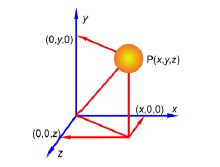We have already looked at the solution for the rotation around the z axis in above figure, Now anticlockwise rotation about the x axis by an angle θ is.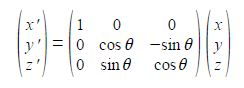and anticlockwise rotation about the y-axis by an angle θ is,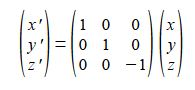Reflection in the xy plane only changes the z coordinate to —z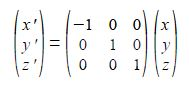and reflection in the yz plane only changes the x coordinate to —x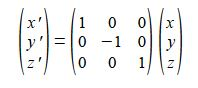and the final reflection, in the zx plane only changes they coordinate to —y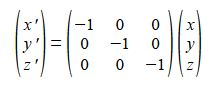Inversion through the origin will change all three coordinates to their negative values